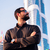##### Sam Alex
5
2023
Mar 15, 2023 09:54 AM

# If I paid 200 to buy a product, how can I calculate VAT on it?

If I paid 200 to buy a product, how can I calculate VAT on it?

All Replies (5)##### Tyche FD
2 months ago

To calculate VAT on a AED 200 product, multiply the purchase price by the VAT rate, usually 5%. So, 200 * 0.05 = AED 10. The VAT amount is AED 10. Add this to the product price, and you'll pay a total of AED 210, including VAT.##### Nandana Nithiyanandan
2 months ago

You paid 200. If 200 is the product value, then 5% vat on it will be 10. So total invoice value inclusive of tax is 210##### Josna Thomas
2 months ago

The VAT amount would be determined using the following formula if you purchased aed 200 for a product: 200 x 5% = 10. Therefore, your total payment, including VAT, would be 200 + 10 = 210.##### Jenifer. Lovely
2 months ago

1. Find out the VAT rate: First, you need to know the VAT rate applicable in your area. VAT is a tax added to the price of goods and services. Let's say the VAT rate is 5%.

2. Calculate the VAT amount: Take the price of the product, which is 200, and multiply it by the VAT rate (5%). So, you would multiply 200 by 0.05 (which is the decimal form of 5%), resulting in 10.

3. Determine the total cost: To find the total amount you paid, add the VAT amount (10) to the original price (200). This will give you the total cost of the product, which is 210.

Therefore, if you paid 200 for the product and the VAT rate is 5%, the total cost including VAT would be 210.

Remember, VAT rates can vary depending on your location, so it's essential to know the specific rate applicable in your area.##### Bryce Dsouza
4 months ago

If you paid 200 to buy a product, the VAT amount would be calculated as follows: 200 x 5% = 10. So, the total amount you would pay including VAT would be 200 + 10 = 210.

Related questions
...
...